# Kotlin let, run, also, apply, with

Published on August 3, 2022By Anupam Chugh
Developer and author at DigitalOcean.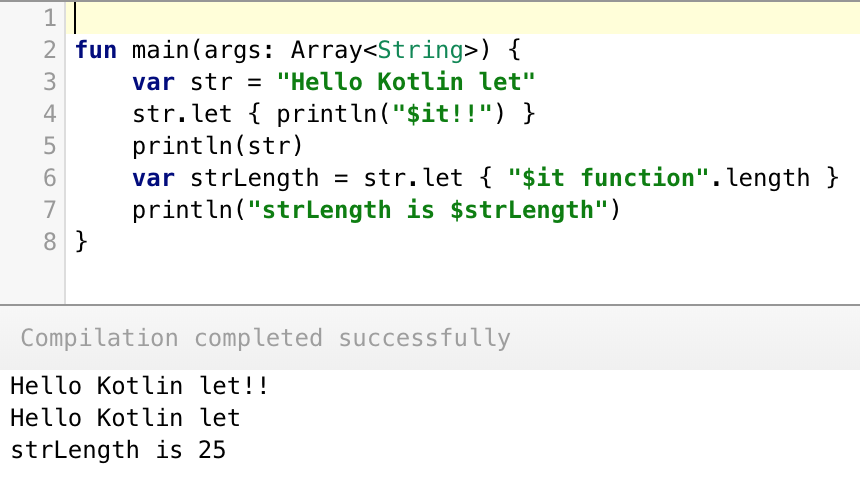While we believe that this content benefits our community, we have not yet thoroughly reviewed it. If you have any suggestions for improvements, please let us know by clicking the “report an issue“ button at the bottom of the tutorial.

In this tutorial, we’ll be implementing some of the important standard library functions available in Kotlin. The `kotlin-stdlib` provides us with useful higher order functions implementing idiomatic patterns. We’ll see how they make programming in Kotlin so easier and faster. The functions that we’re going to discuss below are:

• let
• run
• also
• apply
• with

## Kotlin let

`let` takes the object it is invoked upon as the parameter and returns the result of the lambda expression. Kotlin let is a scoping function wherein the variables declared inside the expression cannot be used outside. An example demonstrating kotlin let function is given below.

``````fun main(args: Array<String>) {
var str = "Hello World"
str.let { println("\$it!!") }
println(str)

}
//Prints
//Hello World!!
//Hello World
``````

`it` keyword contains the copy of the property inside `let`. The last value from the let is returned as an argument as shown below.

``````var strLength = str.let { "\$it function".length }
println("strLength is \$strLength") //prints strLength is 25
``````### Chaining let functions

``````var a = 1
var b= 2

a = a.let { it + 2 }.let { val i = it + b
i}
println(a) //5
``````

As you can see we’ve declared a local variable “i” inside the second let function. Setting the last statement of the let function to `i` returns the property to the outer property `a`.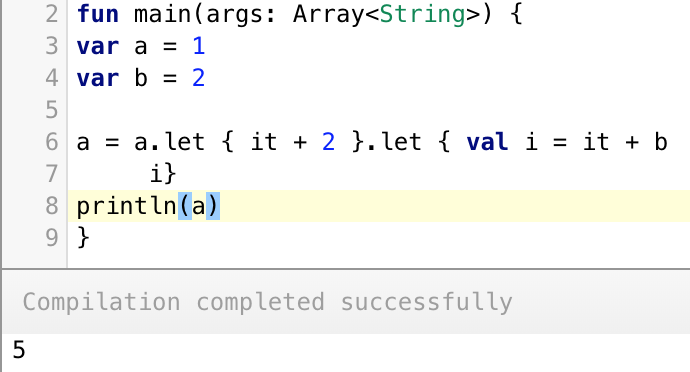### Nesting let

We can set a let expression inside another let expression as shown below.

``````var x = "Anupam"
x.let { outer -> outer.let { inner -> print("Inner is \$inner and outer is \$outer") } }

//Prints
//Inner is Anupam and outer is Anupam
``````

For nested let, we can’t use `it` keyword. We need to assign explicit names to `it` in both the let functions. Only the outermost let returns the value as shown below.

``````var x = "Anupam"
x = x.let { outer ->
outer.let { inner ->
println("Inner is \$inner and outer is \$outer")
"Kotlin Tutorials Inner let"
}
"Kotlin Tutorials Outer let"
}
println(x) //prints Kotlin Tutorials Outer let
``````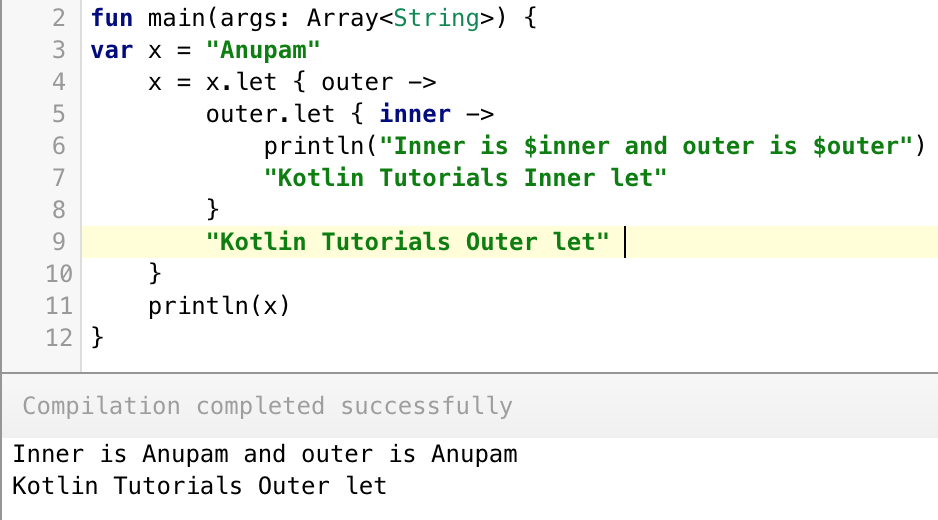### let for null checks

Additionally, let is useful for checking Nullable properties as shown below.

``````var name : String? = "Kotlin let null check"
name?.let { println(it) } //prints Kotlin let null check
name = null
name?.let { println(it) } //nothing happens
``````

The code inside the let expression is executed only when the property is not null. Thus let saves us from the if else null checker too!

## Kotlin run

Kotlin `run` is another interesting function. The following example demonstrates its use cases.

``````var tutorial = "This is Kotlin Tutorial"
println(tutorial) //This is Kotlin Tutorial
tutorial = run {
val tutorial = "This is run function"
tutorial
}
println(tutorial) //This is run function
``````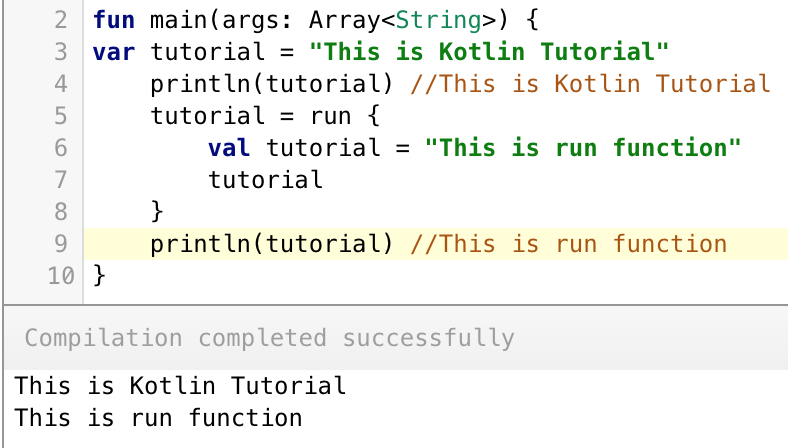Kotlin run expression can change the outer property. Hence in the above code, we’ve redefined it for the local scope.

• Similar to the let function, the run function also returns the last statement.
• Unlike let, the run function doesn’t support the `it` keyword.

### let and run

Let’s combine the let and run functions together.

``````var p : String? = null
p?.let { println("p is \$p") } ?: run { println("p was null. Setting default value to: ")
p = "Kotlin"}

println(p)
//Prints
//p was null. Setting default value to:
//Kotlin
``````

## Kotlin also

As the name says, `also` expressions does some additional processing on the object it was invoked. Unlike let, it returns the original object instead of any new return data. Hence the return data has always the same type. Like `let`, `also` uses `it` too.

``````var m = 1
m = m.also { it + 1 }.also { it + 1 }
println(m) //prints 1
``````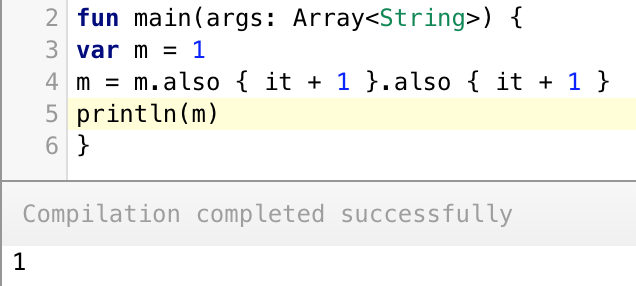### Kotlin let vs also

Following code snippet shows a great example to differentiate between `let` and `also`.

``````data class Person(var name: String, var tutorial : String)
var person = Person("Anupam", "Kotlin")

var l = person.let { it.tutorial = "Android" }
var al = person.also { it.tutorial = "Android" }

println(l)
println(al)
println(person)
``````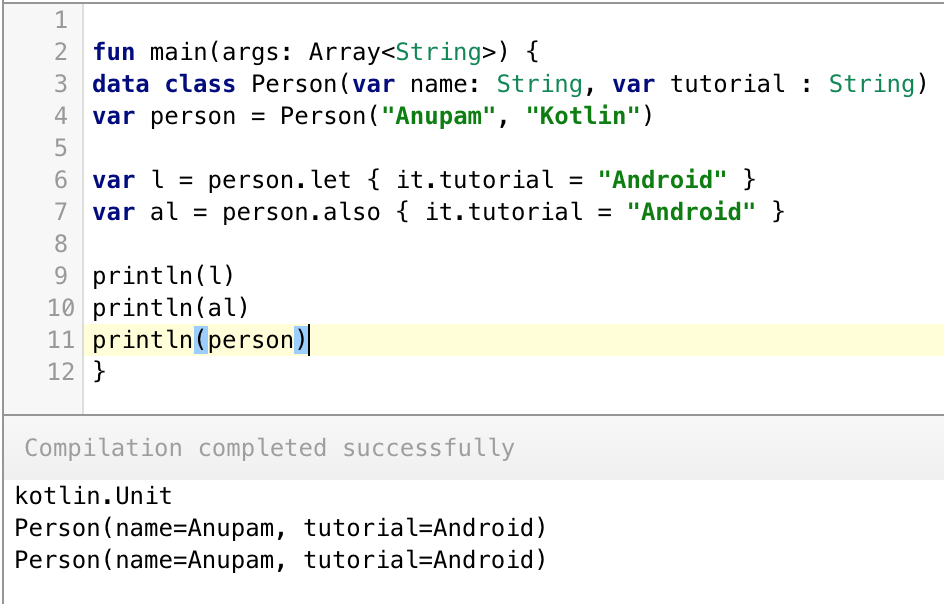In the above code, we’ve used Data classes. The also expression returns the data class object whereas the let expression returns nothing (Unit) as we didn’t specify anything explicitly.

## Kotlin apply

Kotlin `apply` is an extension function on a type. It runs on the object reference (also known as receiver) into the expression and returns the object reference on completion.

``````data class Person(var name: String, var tutorial : String)
var person = Person("Anupam", "Kotlin")

person.apply { this.tutorial = "Swift" }
println(person)
``````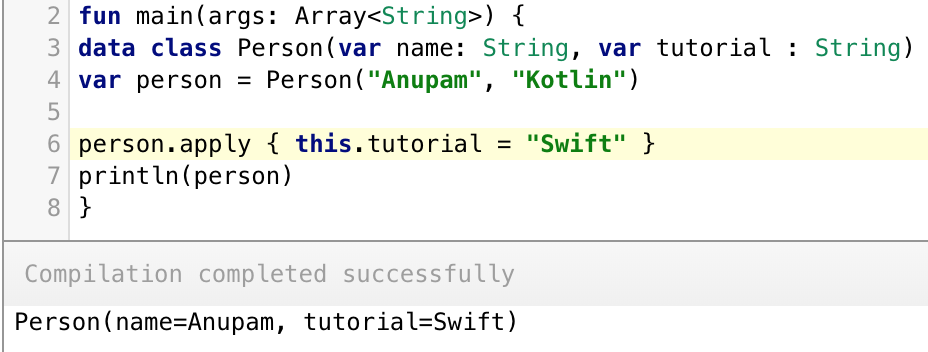### apply vs also

``````data class Person(var n: String, var t : String)
var person = Person("Anupam", "Kotlin")

person.apply { t = "Swift" }
println(person)

person.also { it.t = "Kotlin" }
println(person)
``````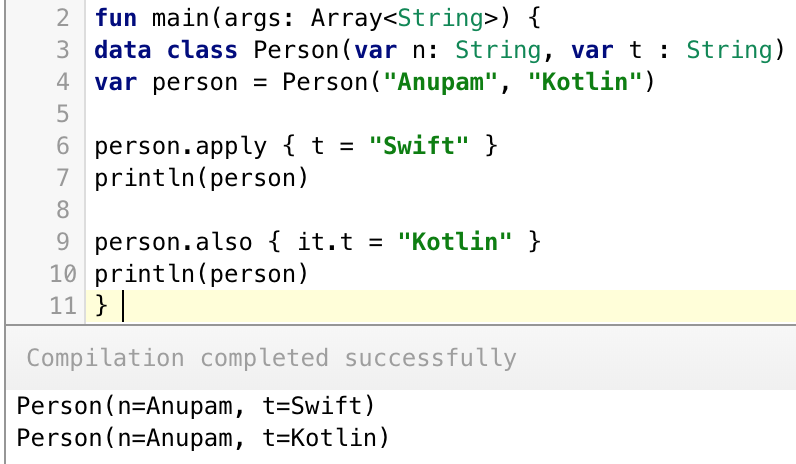Note: In apply `it` isn’t allowed. If the property name of the data class is unique in the function, you can omit `this`. We should use `also` only when we don’t want to shadow `this`.

## Kotlin with

Like `apply`, `with` is used to change instance properties without the need to call dot operator over the reference every time.

``````data class Person(var name: String, var tutorial : String)
var person = Person("Anupam", "Kotlin")

with(person)
{
name = "No Name"
tutorial = "Kotlin tutorials"
}
``````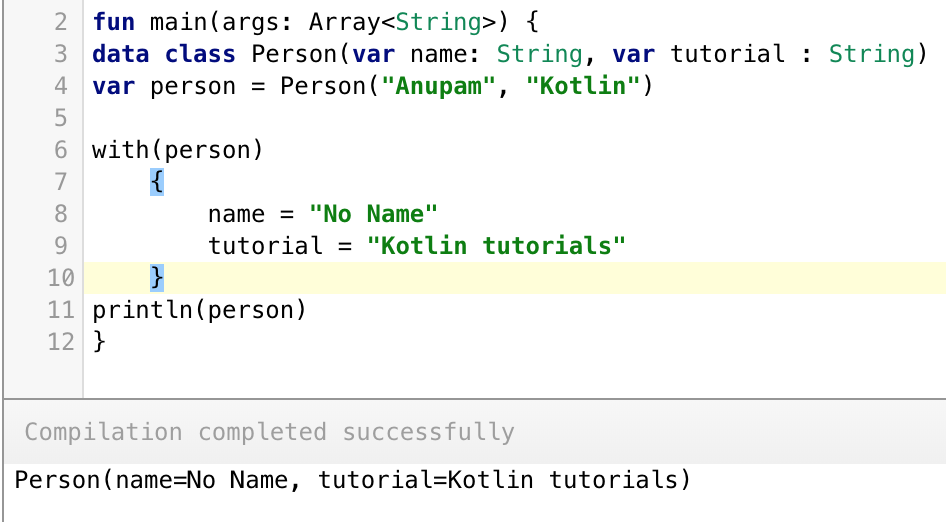Again `with` is similar to `apply` except for a few differences.

### Kotlin apply vs with

• with runs without an object(receiver) whereas apply needs one.
• apply runs on the object reference, whereas with just passes it as an argument.
• The last expression of with function returns a result.
``````var xyz = with(person)
{
name = "No Name"
tutorial = "Kotlin tutorials"
val xyz = "End of tutorial"
xyz
}
println(xyz) //End of tutorial
``````

That’s all for Kotlin standard functions to alter variables or modify objects within the function.Developer and author at DigitalOcean.

#### Still looking for an answer?

let null check code with give you a compile time error if anyone copies and paste it from here… !! Otherwise its very informative. Thanks.

- Soumita

I think it’s the best article I have read on kotlin scoping function. Crystal clear information.

- Astha

Thanks, great examples and good job explaining the differences.

- Gary

Very nice easy to understand each one

- Abhishek Srivastava

Thanks! Very informative and understandable article.

- Anchith

Very clear and specific

- Alif Hasnain

give me some internal mechanism about these functions.

- Nareshkumar